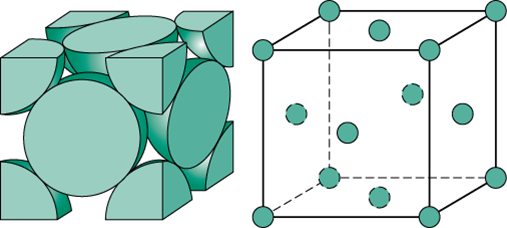#### In a compound, atoms of element Y from ccp lattice and those of element X  occupy 2/3rd  of tetrahedral voids. The formula of the compound will be Option 1)Option 2)Option 3)Option 4)As we learnt in

No. of atoms(z) for face centered unit cell -

Lattice points: at corners and face centers of unit cell.

For face centered cubic (FCC), z=4.

- whereinNumber ofatoms per unit cell inlatticenumber of tetrahedral voidsnumber of tetrahedral voids occupied byHence the formula of the compound will beCorrect option is 2.

Option 1)This is an incorrect option.

Option 2)This is the correct option.

Option 3)This is an incorrect option.

Option 4)This is an incorrect option.

#### divya.saini